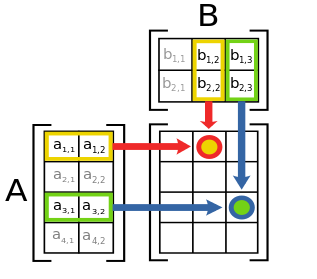# Forecasting Sales and Calculating Revenue - Day 2 of 2

2 teachers like this lesson
Print Lesson

## Objective

SWBAT multiply matrices by a scalar and by another matrix.

#### Big Idea

Matrix multiplication is needed for the Purrrrrfect Cat Toy Company!

## Share and Summarize

30 minutes

The purpose of yesterday’s homework assignment was to see if students could figure out the algorithm for multiplying two matrices together. During yesterday's in-class work we looked at an example of multiplying two matrices in context so students could verify that their graphing calculator was giving them the correct answer when we played around with them. In the homework assignment they did not have a context, but I wanted them to look at other examples to see if they can make any observations about the algorithm for multiplying matrices.

I would be willing to bet that someone will come to class today with the algorithm figured out. I would also be willing to bet that some students (maybe many) will come into class without knowing the algorithm. I start class by giving students about 5 minutes to talk about their findings with their table groups. This gives them a chance to bounce ideas off of each other and troubleshoot what they think matrix multiplication is all about.

After this time I will choose a student to share their thoughts with the class. The rest of the class will be the jury to see if they agree or disagree with the student. If no one in the class truly understands the algorithm, it is okay to guide them to it with a little direct instruction.

After the algorithm has been discussed, I will give students a simple example to work on without their graphing calculator. I’ll go around and check that students are on the right track as a formative assessment. I will choose non-square matrices so that afterwards we can discuss the dimensions and why the number of columns in one matrix must equal the number of rows in the other matrix. The diagram below is from the Wikipedia page for matrix multiplication and is a really good visual representation of the process. It also gives a visual reason why you can’t multiply all matrices together because of the dimensions.The most common thought about multiplying matrices is that you simply multiply corresponding entries in the two matrices to get the new matrix. I will go back to yesterday’s example with the cat toy company to discuss why this is not correct – you could multiply the sales for the East Region and the West region, but that numerical answer would not make any sense. However, multiplying the price by the number of units sold does make sense because it gives us the revenue.

## Extend

20 minutes

Here is the homework assignment I give my students after our summary of matrix multiplication. This assignment definitely ups the ante as student must set up the two matrices to multiply together and then interpret the results. I talk more about the assignment in the video below.

Unable to display content. Adobe Flash is required.

There should be time to start this assignment in class, so I circulate and help students get started but without giving away too much. If a student gets stuck on question #1 for example, you can ask them what specifically needs to be multiplied together to produce the revenue matrix. Students will know that 0.7 must be multiplied by \$14.99 and 1.4 must be multiplied by \$7.99. They can play around with the matrices so that when they perform the algorithm they are getting the same products. This is why presenting in context is so powerful – the checks and balances are built in!